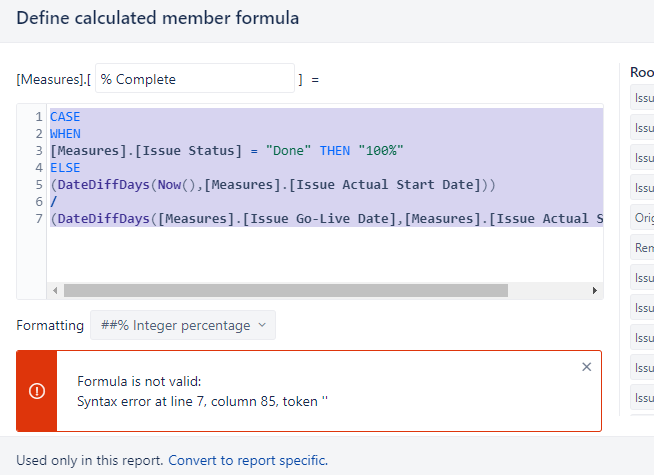# % Complete Between Two Dates

Hi folks.
I want to calculate the percent complete between two dates as of today’s date.

The following is giving me an error:

(Now() - [Measures].[Issue Actual Start Date])
/
([Measures].[Issue Go-Live Date] - [Measures].[Issue Actual Start Date])

Formula is not valid:
No function matches signature ’ - '

Attaching an image also. Unsure if it will display.

Hi @slowlife999,
To get days between two dates you should use the function DateDiffDays().

The syntax is:
`DateDiffDays(from_date, to_date)`

The formula would look something like this:
`DateDiffDays([Measures].[Issue Actual Start Date], Now())`

Kind regards,
Gerda // support@eazyBI.com

Thank you Gerda.

Using the above syntax, I was able to calculate the percentage complete between two dates.

I’m looking now to calculate the percentage complete between two dates if the issue status is in progress and if it’s Done then it should be 100%. However my following Syntax is causing an error. What am I doing incorrectly? Thanks!

CASE
**WHEN **
[Measures].[Issue Status] = “Done” THEN "100%"
ELSE
(DateDiffDays(Now(),[Measures].[Issue Actual Start Date]))
/
(DateDiffDays([Measures].[Issue Go-Live Date],[Measures].[Issue Actual Start Date]))Hi @slowlife999,
Try this formula:

1. It has the closure “END” for the CASE WHEN expression
2. It returns 1 when the status is “Done”
3. It has the val() in case the expression doesn’t recognize the value returned after ELSE
``````CASE WHEN
[Measures].[Issue Status] = "Done"
THEN 1
ELSE
val((DateDiffDays(Now(),[Measures].[Issue Actual Start Date]))
/
(DateDiffDays([Measures].[Issue Go-Live Date],
[Measures].[Issue Actual Start Date])))
END
``````

best,
Gerda

1 Like